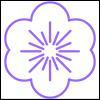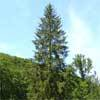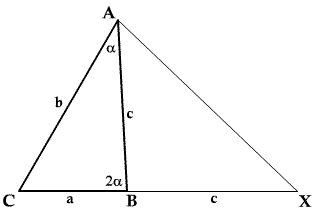#### You may also like### Flower

Six circles around a central circle make a flower. Watch the flower as you change the radii in this circle packing. Prove that with the given ratios of the radii the petals touch and fit perfectly.### Two Trees

Two trees 20 metres and 30 metres long, lean across a passageway between two vertical walls. They cross at a point 8 metres above the ground. What is the distance between the foot of the trees?### Golden Triangle

Three triangles ABC, CBD and ABD (where D is a point on AC) are all isosceles. Find all the angles. Prove that the ratio of AB to BC is equal to the golden ratio.

# Double Angle Triples

##### Age 16 to 18 Challenge Level:Consider the triangle $ABC$ as shown in the diagram. Show that if $\angle B = 2 \angle A$ then $b^2=a^2+ac$. Find integer solutions of this equation (for example, $a=4$, $b=6$ and $c=5$) and hence find examples of triangles with sides of integer lengths and one angle twice another.